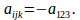## The Single Parameter For Skew Systems of Third Order

Theorem

If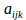is a third order skew symmetric system then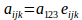where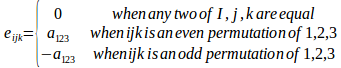Proof

For a skew symmetric matrix,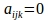if any two indices are equal, and interchanging any two indices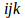changes the sign so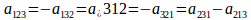If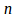is the number of permutations then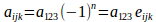where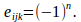Ifis even then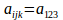and ifis odd then